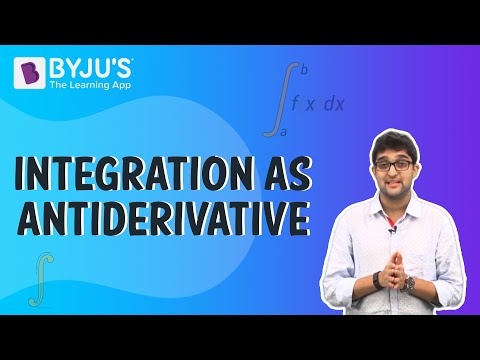# Integrals Class 12 Notes Chapter 7

## Integrals

Integration is referred to the process which is inverse to that of differentiation. In the integration, we will be responsible for finding the function whose differential is provided to us. Integrals are the functions which satisfy a given differential equation.

#### For more information on Integration and Integrals, watch the below video.### What are Indefinite Integrals?

If integration is inverse of differentiation, then

$$\begin{array}{l}\frac{\partial }{\partial x}F(x)=f(x).\; Then \int f(x)dx=F(x) + C\end{array}$$
and these are referred as indefinite or general integrals.are all these differ by a constant term. If seen from a geometrical perspective, an indefinite integral is a compilation of curves which are obtained by translation of one of the curves parallel to itself downward and upward along with y-axis.

### Properties of Indefinite Integrals

Integrals follow certain properties as follows:

• $$\begin{array}{l}\int [f(x)+g(x)dx]=\int f(x)dx+\int g(x)dx\end{array}$$
• For any given real number r,
$$\begin{array}{l}\int r f(x)dx]=r\int f(x)dx\end{array}$$
• If f1, f2, f3 … are the functions and r1,r2,r3… are the designated real numbers. Then,
$$\begin{array}{l}\int [r_{1}f_{1}(x)+r_{2}f_{2}(x)+…+r_{n}f_{n}(x)]dx=r_{1}\int f_{1}(x)dx+r_{2}\int f_{2}(x)dx+…+r_{n}\int f_{n}(x)dx\end{array}$$

### Integration using Partial Fractions

The rational function property can be seen in fractions and is followed by the ratio of two polynomials such as R(x)/S(x) where x and S(x)

$$\begin{array}{l}\neq\end{array}$$
0. If R(x) degree is higher than S(x), then we should divide R(x) by S(x) so that R(x)/S(x)=T(x)+
$$\begin{array}{l}R_{1}(x)/S(x)\end{array}$$
, where T(x) is a degree x polynomial and the degree of
$$\begin{array}{l}R_{1}(x)\end{array}$$
is less than that of S(x). T(x) can be easily integrated.

### Integration using Substitution

In this method, we change the variable of another variable in order to reduce the integral into fundamental integrals. We can get some standard integrals as the following.

• $$\begin{array}{l}\int tan x dx=log\left | secx \right |+C\end{array}$$
• $$\begin{array}{l}\int cot x dx=log\left | sinx \right |+C\end{array}$$
• $$\begin{array}{l}\int sec x dx=log\left | sec x + tan x\right |+C\end{array}$$
• $$\begin{array}{l}\int cosec x dx=log\left | cosec x – cot x\right |+C\end{array}$$
 Also Access NCERT Solutions for Class 12 Maths Chapter 7 NCERT Exemplar for Class 12 Maths Chapter 7

### Important Questions:

 Substitution Method and Simultaneous Equations Integration

## Frequently asked Questions on CBSE Class 12 Maths Notes Chapter 7 Integrals

Q1

### What is calculus?

Branch of mathematics concerned with the calculation of instantaneous rates of change (differential calculus) and the summation of infinitely many small factors to determine some whole (integral calculus).

Q2

### What are the real life uses of integrals?

In real life, integrations are used in various fields such as engineering, where engineers use integrals to find the shape of building.

Q3

### What are the uses of differential calculus?

1. Statistics 2. Data evaluation 3. Credit card/debit card calculations 4. Space technology 5. Mechanical/civil engineering industries